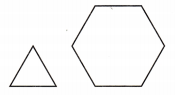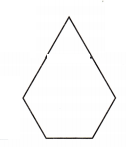# Texas Go Math Grade 2 Lesson 14.3 Answer Key Compose Two-Dimensional Shapes

Refer to our Texas Go Math Grade 2 Answer Key Pdf to score good marks in the exams. Test yourself by practicing the problems from Texas Go Math Grade 2 Lesson 14.3 Answer Key Compose Two-Dimensional Shapes.

## Texas Go Math Grade 2 Lesson 14.3 Answer Key Compose Two-Dimensional Shapes

Explore

Make the shape with pattern blocks. Draw and color the blocks you used.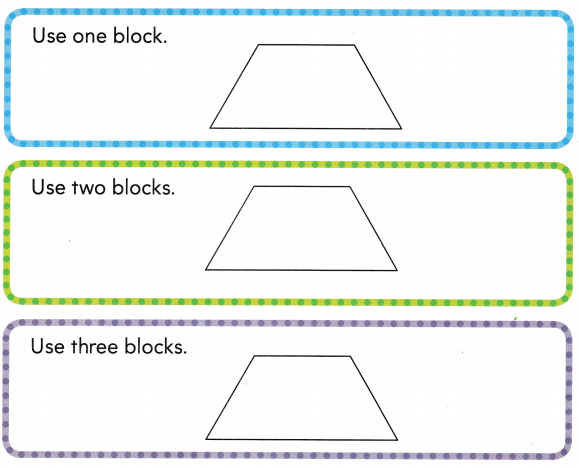FOR THE TEACHER • Read the following problem. Pam uses blocks to make this quadrilateral three different ways. Each time she uses a different number of blocks. What are three ways Pam can make the quadrilateral?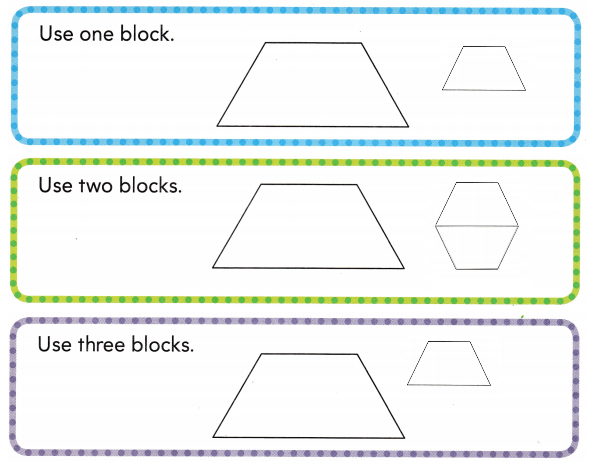Math Talk
Mathematical Processes

Describe the quadrilateral that Pam makes.

Model and Draw

Combine the shapes to make a new shape that has 4 sides.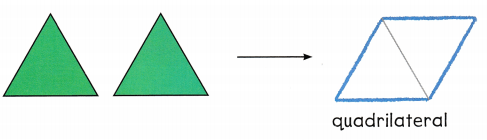Share and Show

Combine the pattern blocks. Trace the blocks to show the new shape you made. Name the new shape.

hexagon
pentagon
octagon
triangle

Make a new shape that has 3 vertices.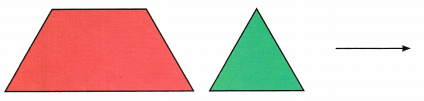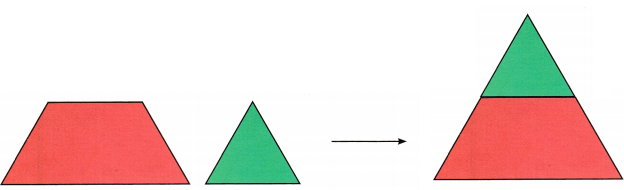Explanation:
A triangle has 3 vertices.

Question 2.
Make a new shape that has 6 sides.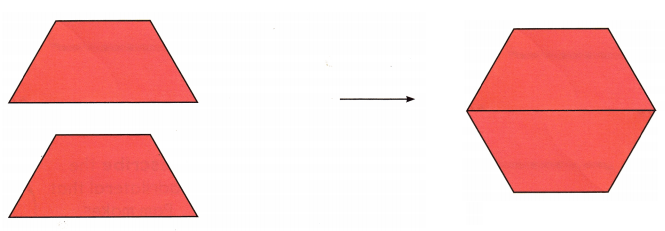Explanation:
A Hexagon has 6 sides.

Problem Solving

Combine the pattern blocks. Trace the blocks to show the new shape you made.

Question 3.
Make a new shape that has more than 6 sides.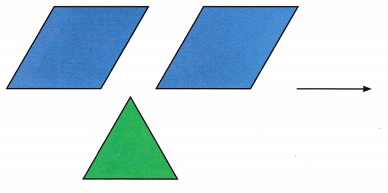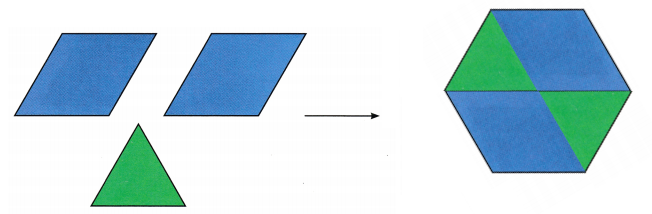Explanation:
I made a hexagon by combining the above shapes.

H.O.T. Todd puts two blocks together to make a pentagon. Trace pattern blocks to show how he could do this.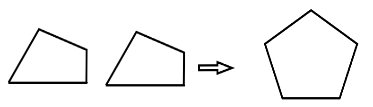Explanation:
Todd makes a pentagon using 2 quadrilaterals.

Question 5.
H.O.T. Multi-Step Sam puts blocks together to make a polygon with 10 sides. Draw to show a polygon she could make. Write the number of vertices.
_________ vertices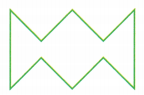Explanation:
Sam puts blocks together to make a polygon with 10 sides.I drew a polygon with 10 sides.A polygon with 10 sides has 10 vertices.

Question 6.
Connect Kim put these two blocks together. What new shape did she make?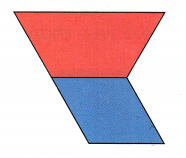(B) pentagon
(C) hexagon
(B) pentagon

Explanation:
If Kim put those 2 blocks together then the new shape has 5 sides. A shape with 5 sides is a pentagon.

Combine the pattern blocks shown. Make a new shape that has 4 sides. Trace the new shape.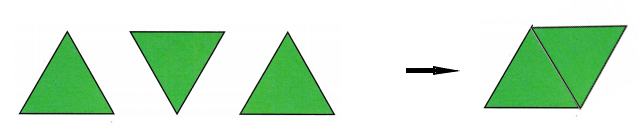Explanation:
If we join 2 triangles we get a quadrilateral as shown above.

Question 8.
Texas Test Prep Shawn put these three blocks together. What new shape did he make?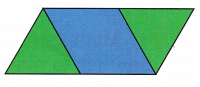(A) pentagon
(B) octagon

Explanation:
The goven shape is a quadrilateral as it has 4 sides.

TAKE HOME ACTIVITY • Have your child explain how he or she solved one of the problems in this lesson.

### Texas Go Math Grade 2 Lesson 14.3 Homework and Practice Answer Key

Question 1.
Combine the pattern blocks to make a new shape that has 5 sides. Draw and name the new shape.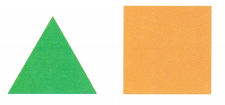Explanation:
A shape that has 5 sides is a pentagon and when we combine the two shapes above we get a new shape with 5 sides.

Problem Solving

Question 2.
Sue put two pattern blocks together to make a quadrilateral. Draw the shape she made.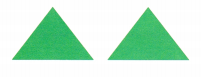Explanation:

Multi-Step Albert put two blocks together to make a polygon with 8 sides. Draw to show a polygon he could make. Write the number of vertices.
__________ verticesExplanation:
Albert put two blocks together to make a polygon with 8 sides. The shape he drew is a octagon. It has 8 sides and 8 vertices.

Lesson Check

Question 4.
Kyle wants to put two shapes together to make a new shape with 4 sides. Which two shapes could Kyle use?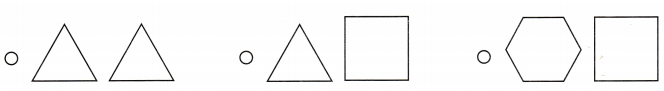Explanation:
Kyle needs to put 2 triangles together to make a shape with 4 sides.
So, i marked the first set of shapes that are triangles.

Explanation:
If kyle wants to put two hapes together that make a new shape with 4 sides kyle could select 2 triangles.

Question 5.
Brenda used two blocks to make a shape with 6 sides. Which shape did she make?
(A) octagon
(B) hexagon
(C) pentagon
(A) Hexagon

Explanation:
If Brenda used two blocks to make a shape with 6 sides.The shape is a hexagon.

Question 6.
Doug put these two shapes together to make a new shape. How many vertices does his new shape have?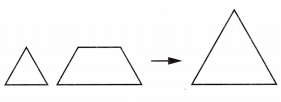(A) 3
(B) 4
(C) 6
(A) 3

Explanation:
If Doug put these two shapes together to make a new shape. The new shape has 3 sides.